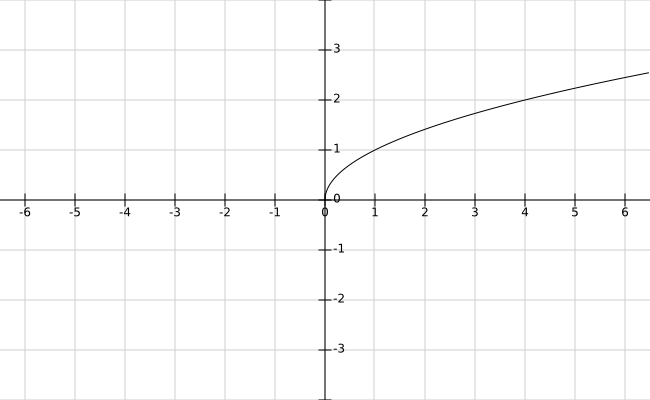# Square Root Function – Graph, Domain and Range

Here you will learn what is square root function with definition, graph, domain and range.

Let’s begin –

## Square Root Function

The function that associates a real number x to +$$\sqrt{x}$$ is called square root function. Since $$\sqrt{x}$$ is real for x $$ge$$ 0. So, we defined the square root function as follows :

Definition : The function f : $$R^+$$ $$\rightarrow$$ R defined by f(x) = +$$\sqrt{x}$$ is called the square root function.

## Square Root Function Graph

The values of f(x) = +$$\sqrt{x}$$ increase with the increase in x.

So, the graph of f(x) = +$$\sqrt{x}$$ is :## Domain and Range :

Clearly, the domain of the square root function is $$R^+$$ i.e [$$0, \infty$$) and its range is also [$$0, \infty$$).

Domain : [$$0, \infty$$)

Range : [$$0, \infty$$)